You are using an outdated browser. Please upgrade your browser to improve your experience.Triangle Calculator computes the area, angles, perimeter, angle bisector and the altitude of a triangle, as well as a diagram of the resulting triangle given with three triangle side input parameters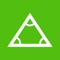Triangle Calculator Plus

by Nitrio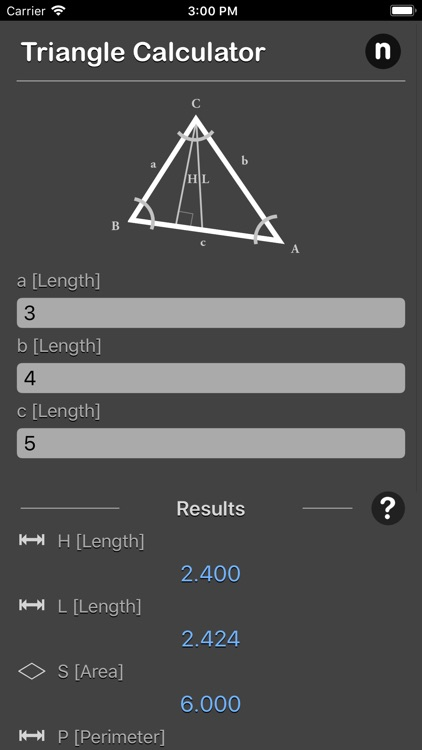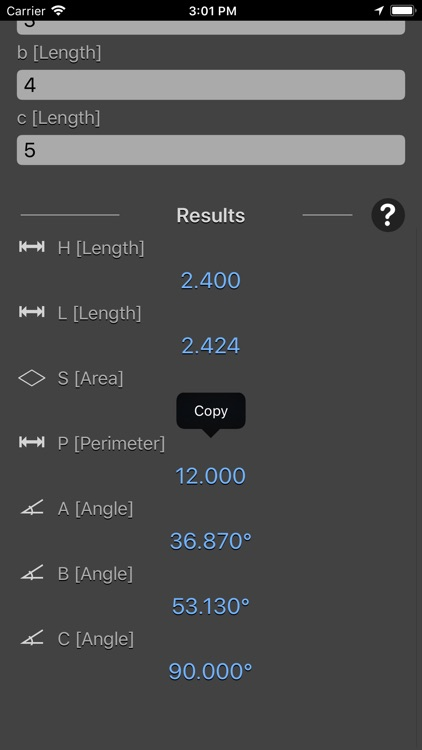What is it about?

Triangle Calculator computes the area, angles, perimeter, angle bisector and the altitude of a triangle, as well as a diagram of the resulting triangle given with three triangle side input parameters.App Details

Version
1.0
Rating
NA
Size
15Mb
Genre
Utilities Productivity
Last updated
June 7, 2018
Release date
May 19, 2018

App Screenshots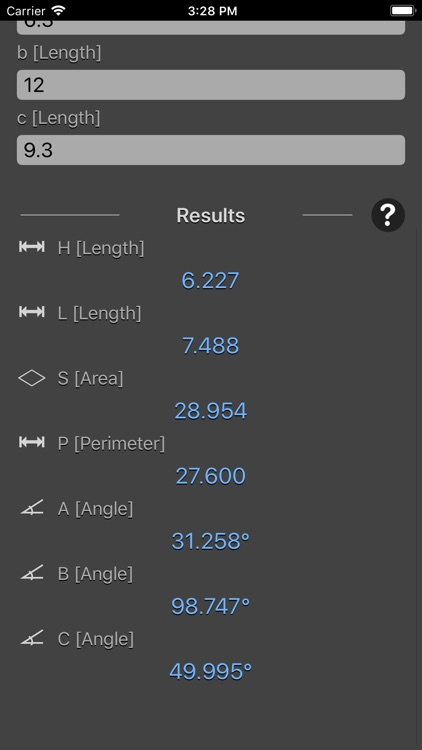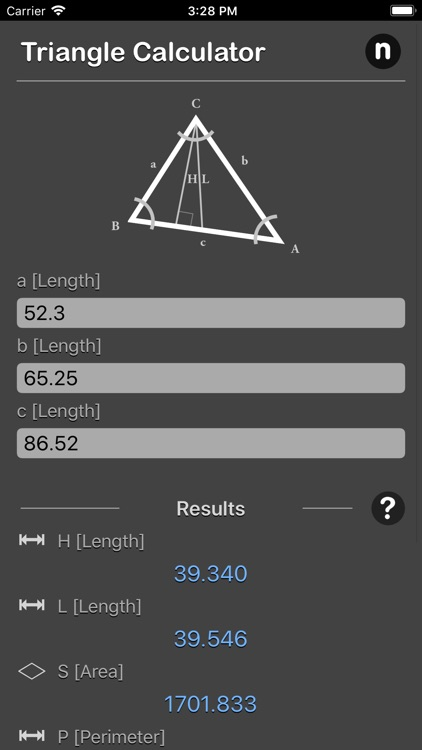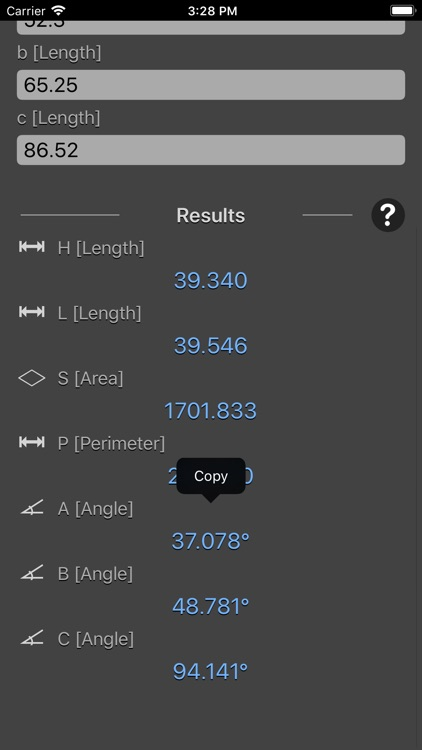App Store Description

Triangle Calculator computes the area, angles, perimeter, angle bisector and the altitude of a triangle, as well as a diagram of the resulting triangle given with three triangle side input parameters.

Triangle vertices are usually named A, B and C. Triangle edges - a, b, c, where letter denotes opposite vertex. That is edge between A and B is named c, between A and C - b, between B and C - a.

The formula are include as reference. For example, S - area of a triangle, which can be found from three known edge by using Hero's formula. All results are show instantly and are copy-able.

A triangle is a polygon that has three vertices. A vertex is a point where two or more curves, lines, or edges meet; in the case of a triangle, the three vertices are joined by three line segments called edges. A triangle is usually referred to by its vertices. Hence, a triangle with vertices a, b, and c is typically denoted as Δabc. Furthermore, triangles tend to be described based on the length of their sides, as well as their internal angles. For example, a triangle in which all three sides have equal lengths is called an equilateral triangle while a triangle in which two sides have equal lengths is called isosceles.

*This is a universal app that work for both iPhone and iPad.

Thanks for your support and do visit nitrio.com for more apps for your iOS devices.

Disclaimer:
AppAdvice does not own this application and only provides images and links contained in the iTunes Search API, to help our users find the best apps to download. If you are the developer of this app and would like your information removed, please send a request to [email protected] and your information will be removed.